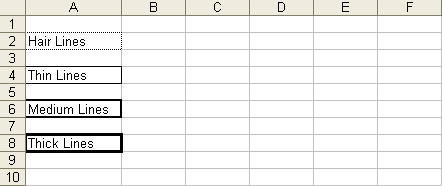# Add Borders to Cells in a Worksheet

Contents
[ ]

To add borders to cells in a spreadsheet, take the following steps:

1. Set up the worksheet:
1. Instantiate an Application object. (VSTO only.)
3. Get the first sheet.
1. Define a range.
2. Apply a border style to the range. Repeat for each range and each border style you want to set. This example applies hairlines, thin, medium and thick lines.
3. Finish:
1. Autofit the column that the cells are in to fit the text neatly.
2. Save the document.

These steps are shown in code below. The first code examples show how to implement them using VSTO with either C# or Visual Basic. After the VSTO examples are examples that show how to perform the same steps using Aspose.Cells for .NET, again using either C# or Visual Basic. The Aspose.Cells code samples are a lot shorter because Aspose.Cells is optimized for efficient coding.

The code generates an Excel file with a number of cells on the first sheet, each with a different border:Cells with borders applied.

C#

`````` .......

using Microsoft.VisualStudio.Tools.Applications.Runtime;

using Excel = Microsoft.Office.Interop.Excel;

using Office = Microsoft.Office.Core;

using System.Reflection;

.......

//Instantiate the Application object.

Excel.ApplicationClass ExcelApp = new Excel.ApplicationClass();

//Get the First sheet.

Excel.Worksheet objSheet = (Excel.Worksheet)objBook.Sheets["Sheet1"];

//Put some text into different cells (A2, A4, A6, A8).

objSheet.Cells[2, 1] = "Hair Lines";

objSheet.Cells[4, 1] = "Thin Lines";

objSheet.Cells[6, 1] = "Medium Lines";

objSheet.Cells[8, 1] = "Thick Lines";

//Define a range object(A2).

Excel.Range _range;

_range = objSheet.get_Range("A2", "A2");

//Get the borders collection.

Excel.Borders borders = _range.Borders;

//Set the hair lines style.

borders.LineStyle = Excel.XlLineStyle.xlContinuous;

borders.Weight = 1d;

//Define a range object(A4).

_range = objSheet.get_Range("A4", "A4");

//Get the borders collection.

borders = _range.Borders;

//Set the thin lines style.

borders.LineStyle = Excel.XlLineStyle.xlContinuous;

borders.Weight = 2d;

//Define a range object(A6).

_range = objSheet.get_Range("A6", "A6");

//Get the borders collection.

borders = _range.Borders;

//Set the medium lines style.

borders.LineStyle = Excel.XlLineStyle.xlContinuous;

borders.Weight = 3d;

//Define a range object(A8).

_range = objSheet.get_Range("A8", "A8");

//Get the borders collection.

borders = _range.Borders;

//Set the thick lines style.

borders.LineStyle = Excel.XlLineStyle.xlContinuous;

borders.Weight = 4d;

//Auto-fit Column A.

objSheet.get_Range("A2", "A2").EntireColumn.AutoFit();

//Save the excel file.

objBook.SaveAs("f:\\test\\ApplyBorders.xls",

Microsoft.Office.Interop.Excel.XlFileFormat.xlExcel8,

Type.Missing,

Type.Missing,

Type.Missing,

Type.Missing,

Microsoft.Office.Interop.Excel.XlSaveAsAccessMode.xlNoChange,

Type.Missing,

Type.Missing,

Type.Missing,

Type.Missing,

Type.Missing);

//Quit the Application.

ExcelApp.Quit();
``````

### Adding Borders using Aspose.Cells for .NET

C#

`````` .......

using Aspose.Cells;

.......

//Instantiate a new Workbook.

Workbook objBook = new Workbook();

//Get the First sheet.

Worksheet objSheet = objBook.Worksheets["Sheet1"];

//Put some text into different cells (A2, A4, A6, A8).

objSheet.Cells[1, 0].PutValue("Hair Lines");

objSheet.Cells[3, 0].PutValue("Thin Lines");

objSheet.Cells[5, 0].PutValue("Medium Lines");

objSheet.Cells[7, 0].PutValue("Thick Lines");

//Define a range object(A2).

Aspose.Cells.Range _range;

_range = objSheet.Cells.CreateRange("A2", "A2");

//Set the borders with hair lines style.

_range.SetOutlineBorders( CellBorderType.Hair, Color.Black);

//Define a range object(A4).

_range = objSheet.Cells.CreateRange("A4", "A4");

//Set the borders with thin lines style.

_range.SetOutlineBorders(CellBorderType.Thin, Color.Black);

//Define a range object(A6).

_range = objSheet.Cells.CreateRange("A6", "A6");

//Set the borders with medium lines style.

_range.SetOutlineBorders(CellBorderType.Medium, Color.Black);

//Define a range object(A8).

_range = objSheet.Cells.CreateRange("A8", "A8");

//Set the borders with thick lines style.

_range.SetOutlineBorders(CellBorderType.Thick, Color.Black);

//Auto-fit Column A.

objSheet.AutoFitColumn(0);

//Save the excel file.

objBook.Save("f:\\test\\ApplyBorders.xls");
``````